# # 3 数学归纳法

## # 3.1 数学归纳法

### # 3.1.1 引入

$\forall n\in\mathbb{N}, \sum_{i=0}^{n}i = \frac{n(n+1)}2\tag{1}$

$\sum_{i=0}^{k}i + (k+1) = \frac{k(k+1)}2 + (k+1) = \frac{(k+1)(k+2)}2\tag{2}$

$\forall n\in\mathbb{N},\sum_{i=0}^{n}i=\frac{n(n+1)}2$

$\sum_{i=0}^{k+1}i = \sum_{i=0}^{k} i + (k+1) = \frac{k(k+1)}2 + (k+1) = \frac{(k+1)(k+2)}2\tag{3}$

### # 3.1.2 归纳

1. 基础情况(Base Case) ：证明 $P(0)$ 为真；
2. 归纳假设(Induction Hypothesis) ：对于某个任意的 $k\ge 0$ ，假设 $P(k)$ 是真的；
3. 归纳步骤(Inductive Step) ：基于归纳假设，证明 $P(k+1)$ 也是真的。### # 3.1.3 实例

#### # 费马小定理

$\forall n\in\mathbb{N}, 3 | (n^3 - n)$

$3 | k^3 - k \wedge 3 | 3k(k+1)$ ，所以 $3 | (k + 1)^3 - (k + 1)$ 。（定理2.1）

#### # 双色定理$P(n)$ 表示语句“任意一张上述形式的有n条线的地图是可二染色的”，有 $\forall n\in\mathbb{N} P(n)$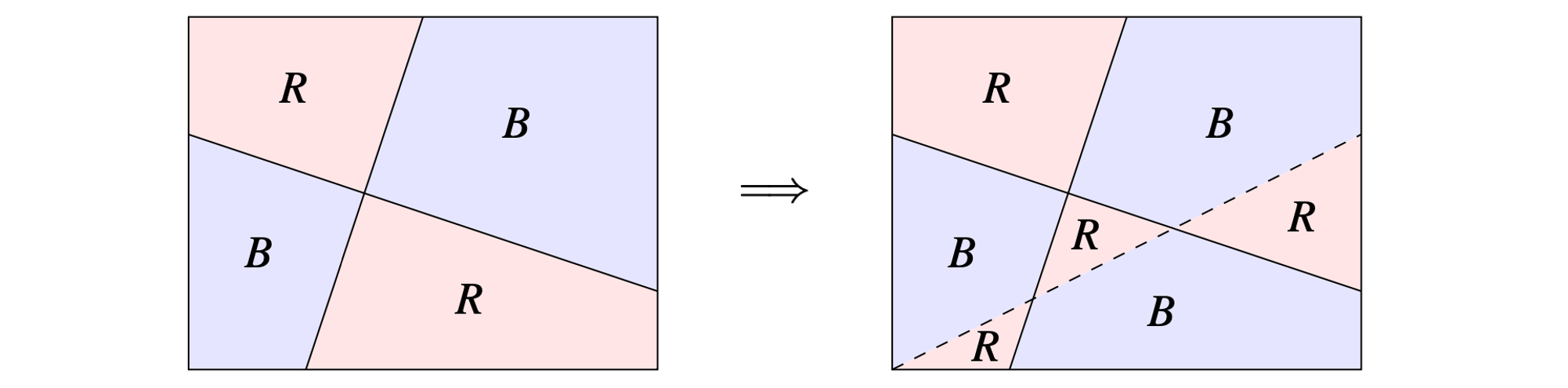• 公共边是我们刚刚移除然后又放回去的那条线，即第 $k + 1$ 条线。
• 根据前面的构造过程，这条线虽然一开始两边的颜色是相同的，但我们将这条线的一侧颜色全部翻转了，因此现在的这两个区域颜色肯定不同；
• 公共边是前 $k$ 条线里面的某一条。
• 由归纳假设以及前面关于翻转颜色的一个基本事实可知，两个区域的颜色肯定也不一样。

## # 3.2 强化归纳假设

### # 3.2.1 引入

• $n = 1$$1 = 1^2$ 是完全平方数。
• $n = 2$$1 + 3 = 4 = 2^2$ 是完全平方数。
• $n = 3$$1 + 3 + 5 = 9 = 3^2$ 是完全平方数。
• $n = 4$$1 + 3 + 5 + 7 = 16 = 4^2$ 是完全平方数。

### # 3.2.3 实例

$\forall n\ge 1, \sum_{i=1}^n\frac1{i^2}\le 2-\frac1n$

$\sum_{i=1}^{k+1}\frac1{i^2} = \sum_{i=1}^{k}\frac1{i^2} + \frac1{(k+1)^2} \le 2 - \frac1k + \frac1{(k+1)^2}$
$= 2 - \frac1{k+1} - \frac1{k(k+1)^2} \le 2 - \frac1{k+1}$

## # 3.3 简单归纳法与强归纳法

### # 3.3.1 概念

• 简单归纳法假设 $P(k)$ 为真，就像我们先前一直做的那样；

• 强归纳法假设一个更强的语句：$P(0), P(1), ..., P(k)$ 都是真的，即

$\bigwedge_{i=0}^{k}P(i)\ is\ true$

• 弱归纳是在说如果第k个骨牌倒了，第k+1个骨牌也会倒；
• 强归纳是在说如果第0个到第k个骨牌都倒了，那么第k+1个骨牌也会倒。

### # 3.3.2 实例

$\forall n\in\mathbb{N}\wedge n\ge 12,\exists x,y\in\mathbb{N}, n=4x+5y$

$\exists x',y'\in\mathbb{N}, (k+1)-4 = 4x' + 5y'$

$x = x' + 1, y = y'$，则

$k + 1 = 4x' + 5y' + 4 = 4(x'+1) + 5y' = 4x+5y$

• $k + 1$ 为质数，则 $P(k+1)$ 显然成立。
• $k + 1$ 不是质数，则 $\exists x,y\in\mathbb{N}, 2 \le x,y \le k, k + 1 = xy$，由归纳假设有 $P(x)\wedge P(y)$ ，而 $k + 1 = xy$ ，于是 $P(k+1)$ 成立。

## # 3.4 递归、编程与归纳法

### # 3.4.1 斐波那契的兔子

13世纪有一位非常著名的意大利数学家斐波那契，他在1202年思考了下面的这个谜题：从一对兔子开始，如果每对兔子从第二个月开始的每个月都能生下一对新兔子（言下之意就是这对兔子刚刚出生的那个月是没有生育能力的），那么一年之后会有多少对兔子呢？这个人口数量增长的模型可以通过递归地定义一个函数来刻画，它的结果数列现在被称为 斐波那契数列(Fibonacci numbers)

• 显然 $F(0) = 0$
• 在第一个月，我们手动引入了第一对新生的兔子，意味着 $F(1) = 1$
• 由于新生的兔子要经过一个月之后才能有生育能力，因此第二个月依然只有一对兔子，即 $F(2) = 1$

• 在第 $n - 1$ 个月，根据定义，我们有 $F(n-1)$ 对兔子。那么，这些兔子中有多少室友生育能力的呢？
• 只有在第 $n - 2$ 个月就已经出生的兔子在第 $n-1$ 个月才有生育能力，也就是说在 $F(n - 1)$ 对兔子中只有 $F(n - 2)$ 对是具有生育能力的。
• 于是，从第 $n - 1$ 个月到第 $n$ 个月的过程中，出生了 $F(n - 1)$ 对兔子，从而第 $n$ 个月的时候，总共有 $F(n) = F(n - 1) + F(n - 2)$ 只兔子。

• $F(0) = 0$
• $F(1) = 1$
• 对于 $n \ge 2$$F(n) = F(n - 1) + F(n - 2)$

There is no exception to the rule that every organic being increases at so high a rate, that if not destroyed, thet earth would soon be covered by the progeny of a single pair. —— Darwin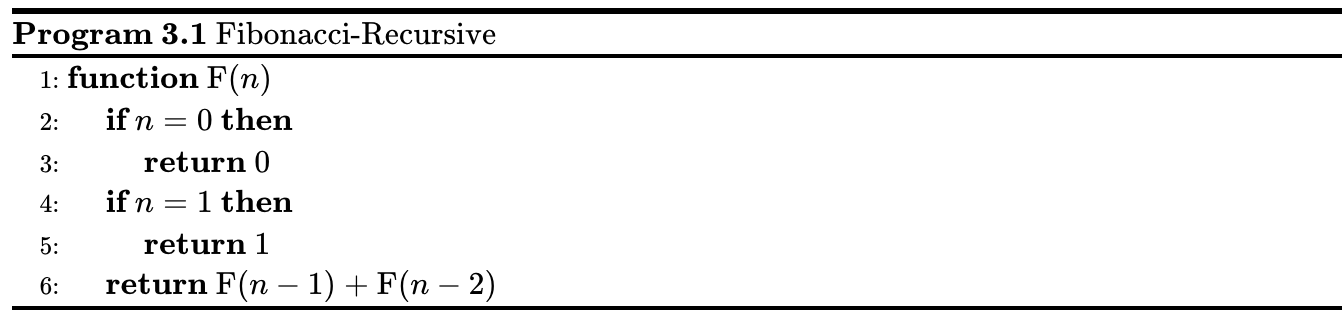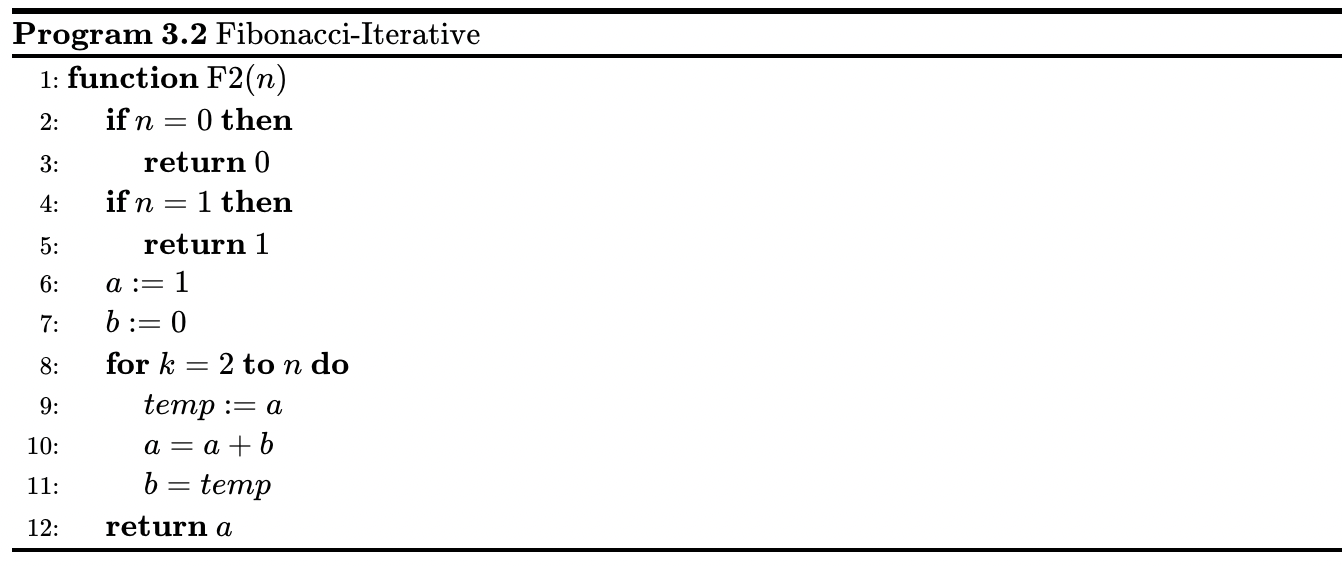### # 3.4.2 二分查找

• 如果这一页的第一个单词在 $W$ 的后面，我们就在字典的前一半（中间页前面的那些页）中继续类似地查找；
• 否则，我们就在字典的后一半（从中间页往后）中继续做类似的查找。
• （如果字典是偶数页，比如说 $2m$ 页，我们定义第 $m + 1$ 页为中间页）
• 一旦我们将查找的范围缩小到一页的时候，我们会在这一页上诉诸于一种暴力的挨个比对的方式来在这一页中查找单词 $W$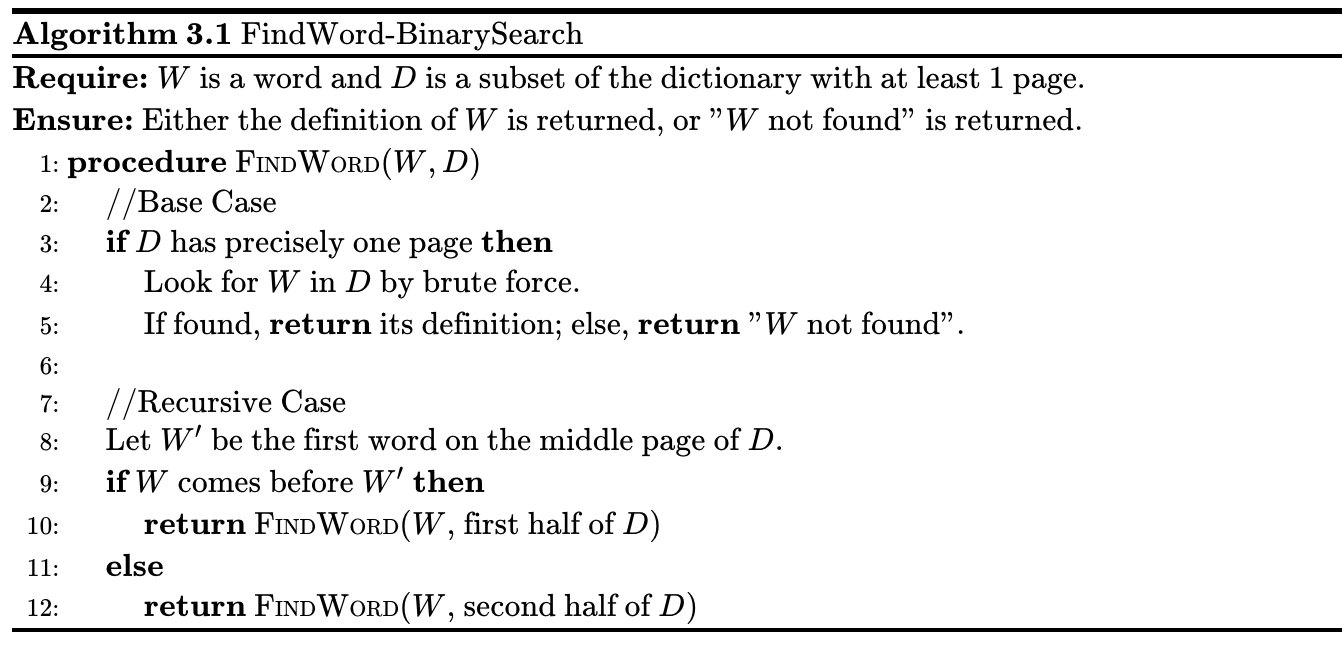## # 3.6 练习题

### # 3.6.1 平方序列的和

$\sum_{i=0}^{k+1}i^2 = \sum_{i=0}^{k}i^2 + (k+1)^2 = \frac16k(k+1)(2k+1) + (k+1)^2$
$=\frac16(k+1)(k(2k+1) + 6(k+1))= \frac16(k+1)(k+2)(2k+3)$

### # 3.6.2 伯努利不等式

$n\in\mathbb{N}\wedge 1+x>0\Longrightarrow (1+x)^n\ge 1+nx$

$(1+x)^{k+1} = (1+x)^k(1+x) \ge (1+kx)(1+x)$
$= 1+(k+1)x + kx^2 \ge 1 + (k+1)x$

### # 3.6.3 阶乘

$\forall n\in\mathbb{N}, n > 1 \Longrightarrow n! < n^n$

$(k+1)! = (k+1)\cdot k! < (k+1)\cdot k^k < (k+1)\cdot (k+1) ^ k = (k+1)^{k+1}$

### # 3.6.4 名人问题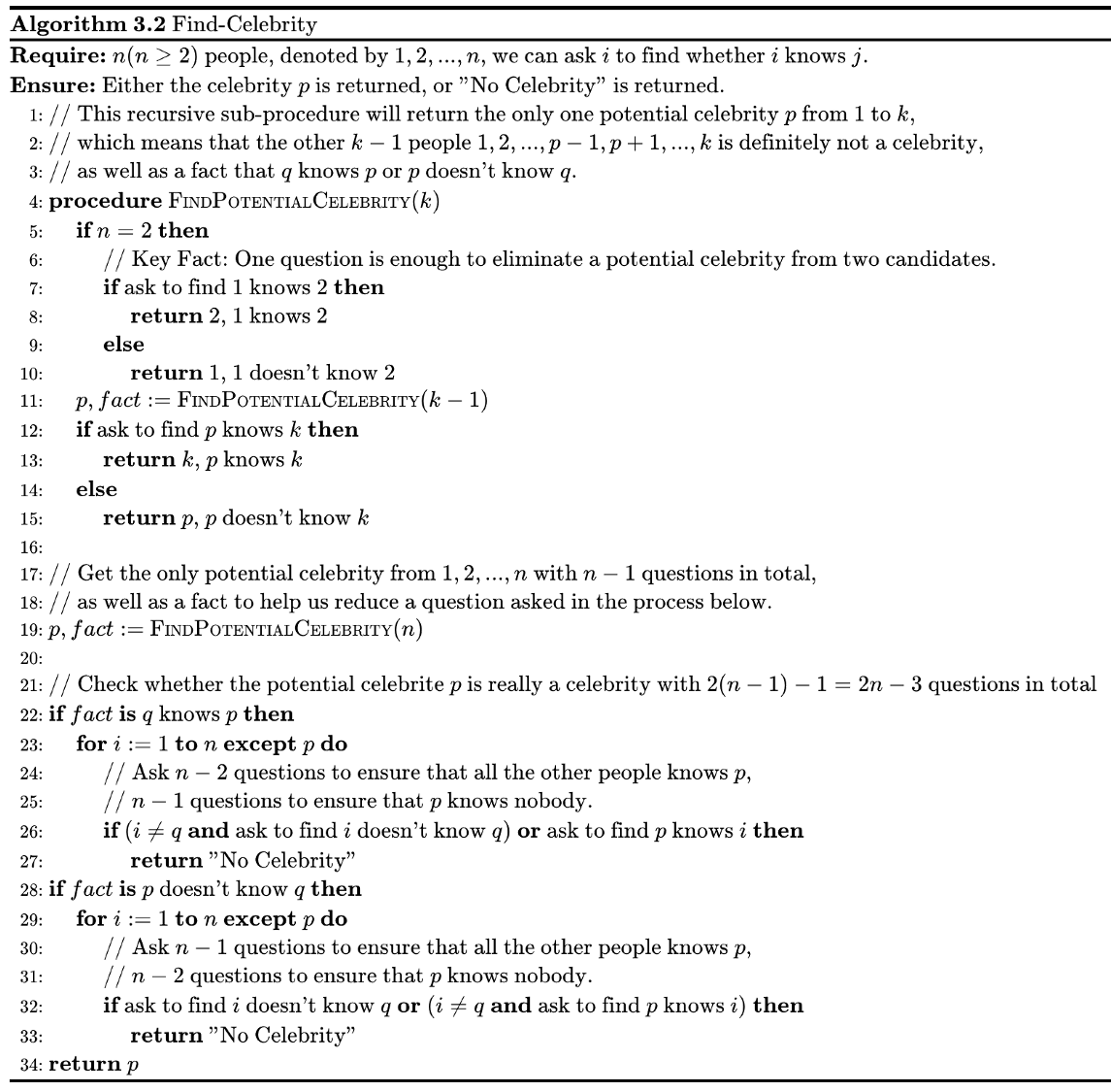$n$ 进行归纳。

• 如果 $p$ 认识 $k$ ，那么 $p$ 一定不是名人，返回 $k$
• 如果 $p$ 不认识 $k$ ，那么 $k$ 一定不是名人，返回 $p$ 。 因此，FindPotentialCelebrity(k) 成功返回了前 $k$ 个人中唯一可能是名人的人，并且只问了 $k - 2 + 1 = k - 1$ 个问题。

### # 3.6.5 邮票兑换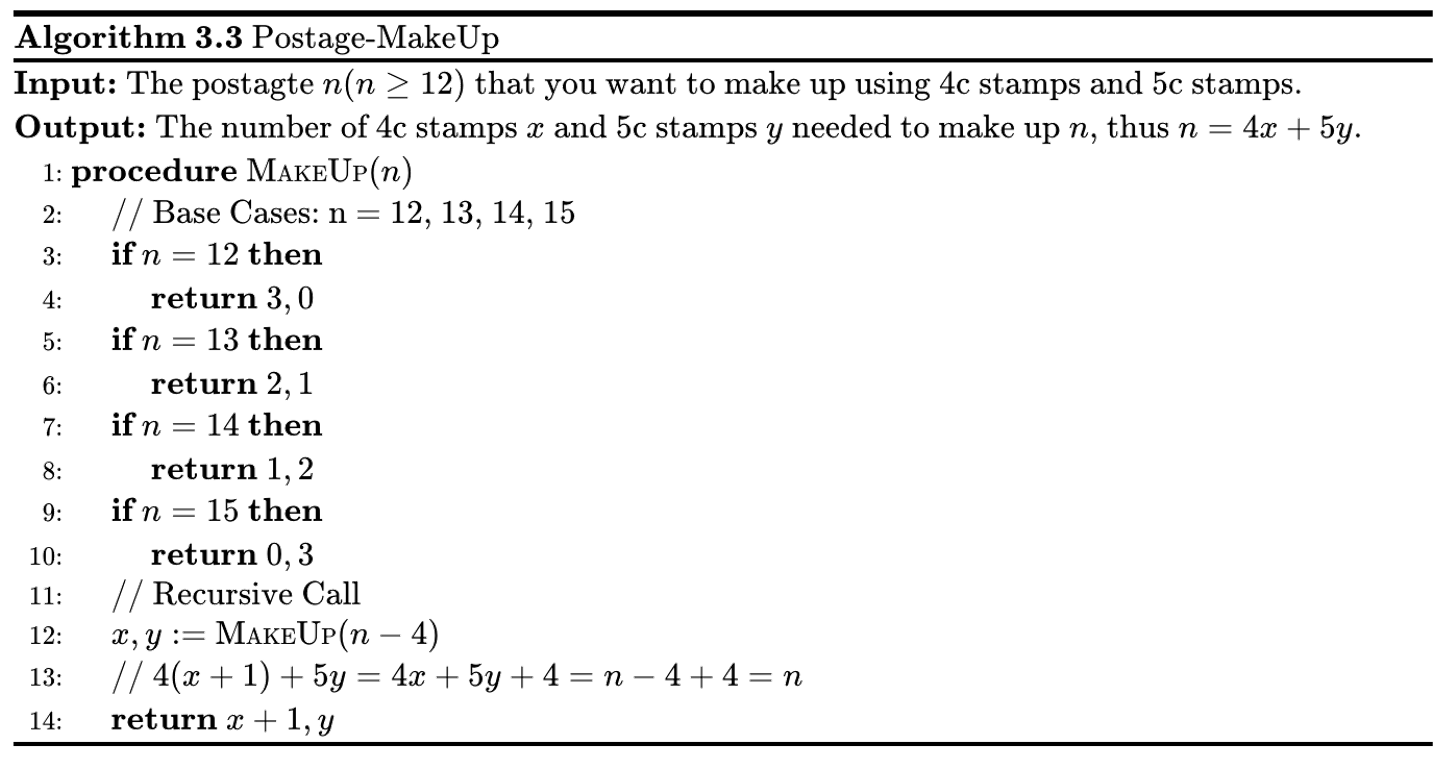$f(n) = \begin{cases} 0, n \equiv 0 \mod 4 \\ 1, n \equiv 1 \mod 4 \\ 2, n \equiv 2 \mod 4 \\ 3, n \equiv 3 \mod 4 \end{cases}, n\ge 12$

### # 3.6.6 强化归纳

$\forall n\in\mathbb{N}^{*}, \sum_{i=1}^{n} \frac1{i^3} \le 2$

$\forall n\in\mathbb{N}^{*}, \sum_{i=1}^{n} \frac1{i^3} \le 2 - \frac1{n^2}$

$\sum_{i=1}^{k + 1} \frac1{i^3} = \sum_{i=1}^{k} \frac1{i^3} + \frac1{(k+1)^3} \le 2 - \frac1{k^2} + \frac1{(k+1)^3}$
$= 2 - \frac1{(k+1)^2} - \frac{k^2 + 3k + 1}{k^2(k+1)^3} \le 2 - \frac1{(k+1)^2}$

$\forall n\in\mathbb{N}^{*}, \sum_{i=1}^{n} \frac1{i^3} \le \sum_{i=1}^{n} \frac1{i^2} \le 2 - \frac1{n} \le 2$

### # 3.6.7 二进制数

$n = c_k\cdot 2^k + c_{k-1}\cdot 2^{k-1} + ... + c_1\cdot 2^1 + c_0\cdot 2^0$

1. $n + 1$ 是偶数，由归纳假设，有
$\frac{n + 1}{2} = c_k\cdot 2^k + c_{k-1}\cdot 2^{k-1} + ... + c_1\cdot 2^1 + c_0\cdot 2^0$
$= \overline{c_kc_{k-1}...c_1c_0}, c_i\in\{0,1\}$

$n + 1 = 2\cdot\frac{n+1}{2} = 2(c_k\cdot 2^k + c_{k-1}\cdot 2^{k-1} + ... + c_1\cdot 2^1 + c_0\cdot 2^0)$
$= c_k\cdot 2^{k+1} + c_{k-1}\cdot 2^{k} + ... + c_1\cdot 2^2 + c_0\cdot 2^1 + 0\cdot 2^0$
$=\overline{c_kc_{k-1}...c_1c_00}, c_i\in\{0,1\}$

$\frac{n}2 = c_k\cdot 2^k + c_{k-1}\cdot 2^{k-1} + ... + c_1\cdot 2^1 + c_0\cdot 2^0$
$= \overline{c_kc_{k-1}...c_1c_0}, c_i\in\{0,1\}$

$n + 1 = 2\cdot\frac{n}{2} + 1 = 2(c_k\cdot 2^k + c_{k-1}\cdot 2^{k-1} + ... + c_1\cdot 2^1 + c_0\cdot 2^0) + 1$
$= c_k\cdot 2^{k+1} + c_{k-1}\cdot 2^{k} + ... + c_1\cdot 2^2 + c_0\cdot 2^1 + 1\cdot 2^0$
$=\overline{c_kc_{k-1}...c_1c_01}, c_i\in\{0,1\}$

## # 3.7 作业题

### # 3.7.1 斐波那契证明

$F_i$ 为第 $i$ 个斐波那契数，由 $F_{i+2} = F_{i+1} + F_i$$F_1 = 1, F_0 = 0$ 。求证：

$\sum_{i=0}^{n} F_i^2 = F_nF_{n+1}$

$\sum_{i=0}^{k + 1} F_i^2 = \sum_{i=0}^{k} F_i^2 + F_{k+1}^2 = F_kF_{k+1} + F_{k+1}^2$
$= F_{k+1}(F_k + F_{k+1}) = F_{k+1}F_{k+2}$